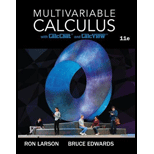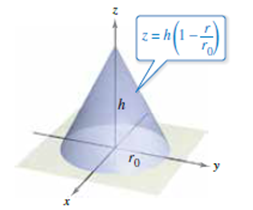# Using Cylindrical Coordinates In Exercises 23-28, use cylindrical coordinates to find the indicated characteristic of the cone shown in the figure. Assume that the density of the cone is ρ ( x , y , z ) = k x 2 + y 2 and find the moment of inertia about the z-axis.### Multivariable Calculus

11th Edition
Ron Larson + 1 other
Publisher: Cengage Learning
ISBN: 9781337275378

#### Solutions

Chapter
Section### Multivariable Calculus

11th Edition
Ron Larson + 1 other
Publisher: Cengage Learning
ISBN: 9781337275378
Chapter 14.7, Problem 28E
Textbook Problem
1 views

## Using Cylindrical Coordinates In Exercises 23-28, use cylindrical coordinates to find the indicated characteristic of the cone shown in the figure.Assume that the density of the cone is ρ ( x , y , z ) = k x 2 + y 2 and find the moment of inertia about the z-axis.

This textbook solution is under construction.

### Still sussing out bartleby?

Check out a sample textbook solution.

See a sample solution

#### The Solution to Your Study Problems

Bartleby provides explanations to thousands of textbook problems written by our experts, many with advanced degrees!

Get Started